# How to find number of days since VLINE.243

Hi there,

I put a VLINE and 2 HLINEs on a chart.

The VLINE is the day a signal occurs that I want to trade.

The HLINE's are profit targets.

I want to calcuate the high or low of the bar I dop the VLINE on because that will be the signal invalidation value. If price reaches it before positions are opened the orders to open should be deleted.

Below is how I'm trying to calculate the number of days since the last server day. I'm only looking at vertical line at the moment.

```for(int k=0;k<ObjectsTotal();k++)
{
Print("Object "+k+": ",ObjectName(k));
if(StringFind(ObjectName(k),"Vertical",0)>=0)
{
ObjectArray[k]=ObjectGet(ObjectName(k),OBJPROP_TIME1);
double SecondsSinceSignal=StringToInteger(TimeCurrent()-ObjectArray[k]);
double MinutesSinceSignal=SecondsSinceSignal/60;
int DaysResult=MathRound(DaysSinceSignal);

Print("Seconds since signal bar: ",SecondsSinceSignal);
Print("Minutes since signal bar: ",MinutesSinceSignal);
Print("Days since signal bar: ",DaysSinceSignal);
Print("DaysResult: ",DaysResult);
}
else
{
ObjectArray[k]=ObjectGet(ObjectName(k),OBJPROP_PRICE1);
}
}//End for loop```

I place a vline on the most right bar with a result of 1. For every bar I move to the left the result increases by 1 until when the result should read 6 but reads 8.

The below captures show the prints from the most right bar and moving left.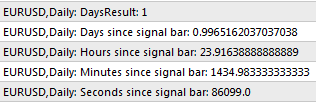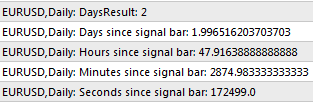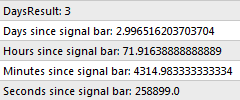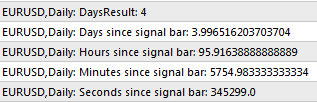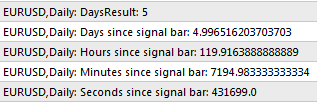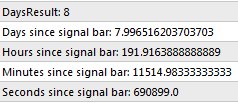Got any idea how to get consistant result or approach the whole thing better?

Thanks.243

"Below is how I'm trying to calculate the number of days since the last server day."

"...number of days from the last/current server day to the day the VLINE is on."3196243

That looks good, will give it a go. Thanks.
qjol:
iBarShift243

Yep, worked a charm. Thanks.6

This function count days left:

int countDaysLeft(datetime expDate){

datetime curDate = TimeCurrent();
string strCurDate = TimeYear(curDate)+"."+TimeMonth(curDate)+"."+TimeDay(curDate);
curDate = StrToTime(strCurDate);

return (int)StringToInteger(expDate-curDate)/(60*60*24);;
}19191

brettosm8: Yep, worked a charm. Thanks.

Except it returns the number of bars ago, not the number of days ago, which was your original question.